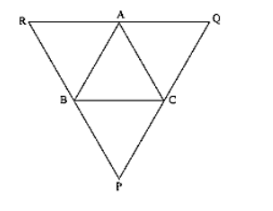# ABC is a triangle and through A, B, C lines are drawn parallel to BC,

Question:

ABC is a triangle and through A, B, C lines are drawn parallel to BC, CA and AB respectively intersecting at P, Q and R. Prove that the perimeter of ΔPQR is double the perimeter of ΔABC.Solution:

Clearly ABCQ and ARBC are parallelograms.

Therefore, BC = AQ and BC = AR

⟹ AQ = AR

⟹ A is the mid-point of QR

Similarly B and C are the mid points of PR and PQ respectively.

∴ AB = (1/2) PQ, BC = (1/2) QR, CA = (1/2) PR

⟹ PQ = 2AB, QR = 2BC and PR = 2CA

⟹ PQ + QR + RP = 2 (AB + BC + CA)

⟹ Perimeter of ΔPQR = 2 (perimeter of ΔABC)